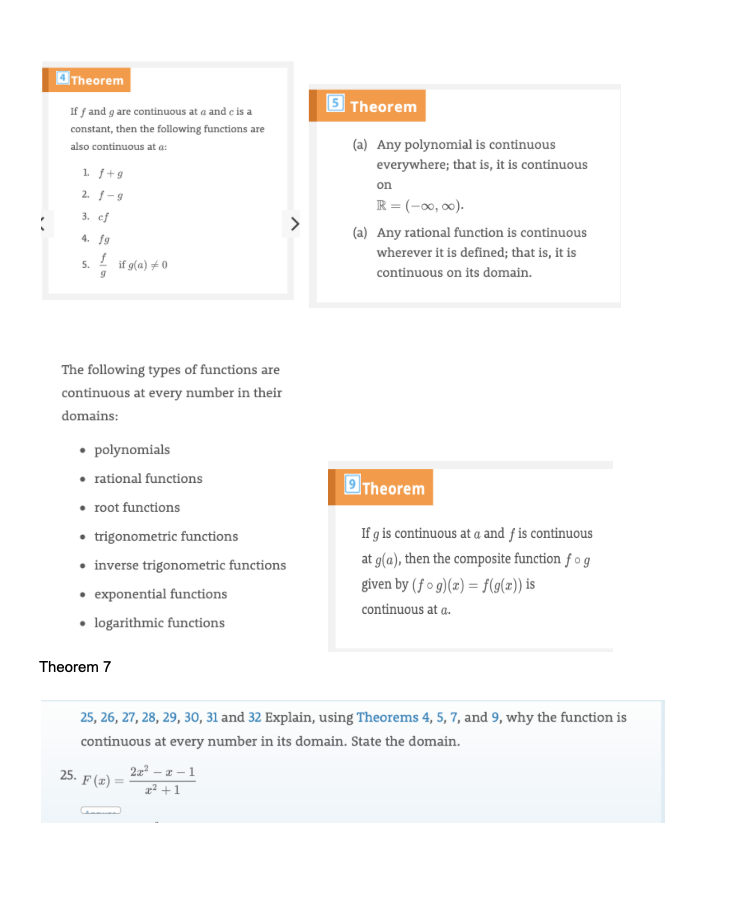# Theorem 5 Theorem If f and g are continuous at a and e is a constant, then the following functions are (a) Any polynomial is continuous also continuous at a: everywhere; that is, it is continuous 1f+9 on 2. -9 TR =(00, 00) 3. ef (a) Any rational function is continuous 4. fg wherever it is defined; that is, it is f if g(a) 0 5. continuous on its domain The following types of functions are continuous at every number in their domains: polynomials rational functions 9 Theorem root functions trigonometric functions inverse trigonometric functions If g is continuous at a and f is continuous at g(a), then the composite function fo g given by (fo g)() = f(g(#)) is exponential functions logarithmic functions continuous at a. Theorem 7 25, 26, 27, 28, 29, 3o, 31 and 32 Explain, using Theorems 4, 5, 7, and 9, why the function is continuous at every number in its domain. State the domain. 25. F(22-2-1 21

Questionhelp_outlineImage TranscriptioncloseTheorem 5 Theorem If f and g are continuous at a and e is a constant, then the following functions are (a) Any polynomial is continuous also continuous at a: everywhere; that is, it is continuous 1f+9 on 2. -9 TR =(00, 00) 3. ef (a) Any rational function is continuous 4. fg wherever it is defined; that is, it is f if g(a) 0 5. continuous on its domain The following types of functions are continuous at every number in their domains: polynomials rational functions 9 Theorem root functions trigonometric functions inverse trigonometric functions If g is continuous at a and f is continuous at g(a), then the composite function fo g given by (fo g)() = f(g(#)) is exponential functions logarithmic functions continuous at a. Theorem 7 25, 26, 27, 28, 29, 3o, 31 and 32 Explain, using Theorems 4, 5, 7, and 9, why the function is continuous at every number in its domain. State the domain. 25. F(22-2-1 21 fullscreen

### Want to see this answer and more?

Experts are waiting 24/7 to provide step-by-step solutions in as fast as 30 minutes!*

*Response times vary by subject and question complexity. Median response time is 34 minutes and may be longer for new subjects.
Tagged in
MathCalculus

### Functions